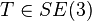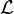# Modern Robotics Errata

(Difference between revisions)

The errata below are for the updated first edition of Modern Robotics (as well as the practice exercises and linear algebra refresher appendix). The updated first edition (also called "version 2") was originally published by Cambridge University Press in late 2019 (marked "3rd printing 2019" or later) and the corresponding online preprint is dated December 2019. The updated first edition includes several corrections and minor additions to the original first edition, which was originally published by Cambridge in May 2017, with a corresponding online preprint dated May 2017.

## Updated first edition: Significant corrections (Errata for original first edition here)

### Chapter 3

• (printed version only) At the end of the introduction Exercise 3.16, it says "origin of {b} is at (0,2,0) is {s}" but "is {s}" should be "in {s}".
• Exercise 3.20, Figure 3.26: In the figure, the y and z axes for the {a}, {b}, and {c} frames are switched (y should point forward and z should point up). Also, the space frame is located at the bottom of the small wheel, directly below the {a} frame.
• (printed version only) Exercise 3.25(a): the element in the third row and third column of the matrix A should be 0 (it is incorrectly written as 1).

### Chapter 4

• Exercise 4.21: The question should begin "For each T below..." (instead of "For each$T \in SE(3)$ below...") since the first part of the problem is determining whether T is indeed an element of SE(3).

### Chapter 8

• (printed version only) Equation (8.74): the first two plus signs should be minus signs.

### Chapter 10

• Second displayed equation of Chapter 10.6.3 (Workspace Potential): As it is written, this equation (which involves a partial derivative with respect to the robot's configuration q) already gives the repulsive generalized force Fij(q), i.e., the Jacobian is already embedded, obviating the subsequent development. To fit the rest of the development, the partial derivative in this equation should be with respect to fi(q). So the equation should read$F^\prime_{ij}(q) = -\frac{\partial P^\prime_{ij}}{\partial f_i(q)} = \frac{k}{\|f_i(q) - c_j\|^4} (f_i(q) - c_j) \in \mathbb{R}^3.$

### Chapter 11

• Chapter 11.5, Equations (11.52) and (11.53) (and nearby text): The term Kfp in Equations (11.52) and (11.53) should be (Kfp + I), where I is the identity matrix. In the text immediately after Equation (11.51), the term "positive-definite" should be eliminated. In the text immediately after Equation (11.53), Kfp should be replaced by (Kfp + I).

## Updated first edition: Minor typos, etc., no danger of misunderstanding (Errata for original first edition here)

### Throughout the book

• The V-REP simulator has been discontinued and replaced by the CoppeliaSim simulator. This does not change anything in the book (or the simulation scenes provided to accompany the book).

### Chapter 2

• Figure 2.9 (left): bold segment of the line should not extend beyond the closing parenthesis at b.

### Chapter 5

• Chapter 5.3, Case V: For maximum clarity, the title should be "Case V: Six Revolute Joint Axes Intersecting a Common Line." Similarly, fifth bullet of Chapter 5.5: item (v) on the list should say "six revolute joint axes intersecting..." instead of just "six revolute joints intersecting..."

### Chapter 6

• (printed version only) Chapter 6.2.2, Example 6.1: just before the matrix Tsd, "corresponds to to" should be "corresponds to."
• (printed version only) Chapter 6.3, first sentence after Equation (6.7): "however small" should be written "however, small" to avoid ambiguity.

### Chapter 8

• First bullet of Chapter 8.10: In the displayed equation, the math italic L should be in the calligraphic font$\mathcal{L}$, for the Lagrangian.

### Chapter 11

• (online version only) Chapter 11.3.3: The sentence containing Equation (11.18) is missing a period at the end.

## A partial list of errata contributors

Thanks to the following people who provided corrections, starting from the preliminary version of the book posted in October, 2016:

H. Andy Nam, Eric Lee, Yuchen Rao, Chainatee Tanakulrongson, Mengjiao Hong, Kevin Cheng, Jens Lundell, Elton Cheng, Michael Young, Jarvis Schultz, Logan Springgate, Sofya Akhmametyeva, Aykut Onol, Josh Holcomb, Yue Chen, Mark Shi, AJ Ibraheem, Yalun Wen, Seongjae Jeong, Josh Mehling, Felix Wang, Drew Warren, Chris Miller, Clemens Eppner, Zack Woodruff, Jian Shi, Jixiang Zhang, Shachar Liberman, Will Wu, Dirk Boysen, Awe Wang, Ville Kyrki, John Troll, Andrew Taylor, Nikhil Bakshi, Yunzhe Pan, Barrett Ames, Marcel Bonnici, Mahdiar Edraki, Jay Li, Jose Capco, Chen Wang